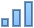# Create scatter charts and bubble charts

Scatter charts display data as points, and they require at least two columns or rows of data to plot values for a single data series. To show multiple data series, you use additional two-column or two-row pairs.

Bubble charts are similar to scatter charts, but they plot data as bubbles. Each data series includes a third dimension that conveys the relationship between the compared values (x and y) and a size value (z). The size value determines the size of the bubble.

There are two ways to create these charts. You can add a blank chart and then select the table cells with the data you want to use. Or, you can select table cells first, then create a chart that displays the data. Either way, when you change the data in the table, the chart updates automatically.

This topic describes how to add a chart then select data.

## Create a scatter chart

1. Click Chartin the toolbar, then select the type of scatter chart you want to add.

Click the 2D or Interactive tab to see all the options; there are no 3D scatter charts.

2. Click Add Chart Data below the chart. If you don’t see Add Chart Data, make sure the chart is selected.

3. Select the table rows or columns with the data you want to use by clicking the numbered or lettered bar for those rows or columns.

You can select rows or columns from one or more tables, including tables on different sheets.

If you select two columns: The scatter chart plots one data series. The first column selected determines the x-axis values and the second column determines the y-axis values.

If you select more than two columns: The first column selected determines the x-axis values and each additional column selected creates a new series (and determines the y-axis values for that series).

To have each scatter series use a different column for the x-axis values, deselect Share X Values from the pop-up menu in the bar at the bottom of the window.

If you select only a single column: The scatter chart plots the data similar to a line chart, with the values from the selected column along the y-axis.

4. To change whether rows or columns are plotted as series, choose an option from the pop-up menu in the bar at the bottom of the window.

5. Click Done.

## Create a bubble chart

Bubble charts use three values to determine each data series. The first two values (x and y) determine the position (or coordinates) of the bubble, and the third value (z) determines the size of the bubble.

1. Click Chartin the toolbar, then select the type of bubble chart you want to add.

Click the 2D or Interactive tab to see all the options; there are no 3D bubble charts.

2. Click Add Chart Data below the chart. If you don’t see Add Chart Data, make sure the chart is selected.

3. Select the table rows or columns with the data you want to use by clicking the numbered or lettered bar for those rows or columns.

You can select rows or columns from one or more tables, including tables on different sheets.

• Plot one data series: Select three columns. The first column selected determines the x-axis values, the second column determines the y-axis values, and the third column determines the size of the bubble.

• Plot more than one data series: Select sets of three columns. The bubble chart plots each group of three columns as one data series. The first column selected determines the x-axis values, the second column determines the y-axis values, and the third column determines the sizes of the bubbles.

To have each bubble series share one column for the x-axis values, choose Share X Values from the pop-up menu in the bar at the bottom of the window. In this case, select sets of two columns for each additional data series.

• Use data from a single column: Select the column. The bubble chart plots the data similar to a line chart, with the values from the selected column along the y-axis.

4. To change whether rows or columns are plotted as series, choose an option from the pop-up menu in the bar at the bottom of the window.

5. Click Done.

## Share the x-axis for multiple values along the y-axis

Sharing the x-axis means plotting a single kind of value along the x-axis, while allowing for multiple kinds of values to be plotted along the y-axis.

1. Select the chart, then click Edit Data References.

2. Choose Share X Values from the pop-up menu in the bar at the bottom of the window.

3. Click Done.

You can change the data ranges that the chart refers to at any time. To learn how, see Modify chart data references.